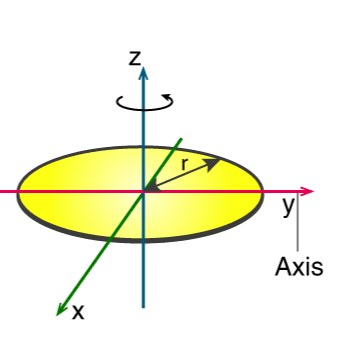Question

In: Computer Science

To calculate Moment of inertia of DISC

explain how to calculate the moment of inertia of a disk, we will take the example of a uniform thin disk which is rotating about an axis through its centre.

Solutions

Expert Solution

Concept.

As we have a thin disk, the mass is distributed all over the x and y plane. Then, we move on to establishing the relation for surface mass density (σ) where it is defined as or said to be the mass per unit surface area. Since the disk is uniform, therefore, the surface mass density will also be constant where;

σ= m / A

or

σA=m

so

dm=σ(dA)

Now it is time for the simplification of the area where it can be assumed the area to be made of a collection of rings that are mostly thin in nature. The thin rings are said to be the mass increment (dm) of radius r which are at equal distance from the axis. The small area (dA) of every ring is further expressed by the length (2πr) times the small width of the rings (dr.) It is given as;

A = πr2, dA = d(πr2) = πdr2 = 2rdr

Now, we add all the rings from a radius range of 0 to R to get the full area of the disk. The radius range that is given is the value that is used in the integration of dr. If we put all these together then we get;

I = ∫r^2σ(πr)dr limit 0-R

I = 2 π σ∫r^3dr

I = 2 πσ r^4 / 4 |. Limit - 0--R

I = 2 πσ (r^4 / 4 – 0)

I = 2 π (m / 4 )(R^4 / 4)

I = 2 π (m / π r^2 )(R4 / 4)

I = ½ mR2.Momemt of inertia of disc at centre -½ MR^2

Related Solutions

To calculate moment of inertia
To calculate moment of inertia of axis passing through centre of a square
To calculate moment of inertia of a system
Two balls connected by a rod as shown in the figure below (Ignore rod’s mass). What is the moment of inertia of the system? Given :  mX = 400 grams = 0.4kg   mY = 500 grams = 0.5 kg   rX = 0cm = 0m   rY = 30cm = 0.3m
Calculate moment of inertia of disc with remaining portion
From a uniform circular disc of radius R and mass 9 M, a small disc of radius R/3 is removed as . Calculate the moment of inertia of the remaining disc about an axis perpendicular to the plane of the disc and passing through the centre of the disc.
Calculate moment of inertia of disc with remaining portion
From a uniform circular disc of radius R and mass 9 M, a small disc of radius R/3 is removed as . Calculate the moment of inertia of the remaining disc about an axis perpendicular to the plane of the disc and passing through the centre of the disc.
Problem on moment of inertia
Two balls connected by a rod, as shown in the figure below (Ignore rod’s mass). Mass of ball X is 8kg and the mass of ball Y is 5 kg  What is the moment of inertia of the system about AB?
moment of inertia of a rod
Starting with the formula for the moment of inertia of a rod rotated around an axis through one end perpendicular to its length (I=M ℓ² / 3), prove that the moment of inertia of a rod rotated about an axis through its center perpendicular to its length is I=M ℓ² / 12. You will find the graphics in Figure 10.12 useful in visualizing these rotations.
Moment of inertia for equilateral triangle
Three rods each of mass m and length I are joined together to form an equilateral triangle as shown in figure. Find the moment of inertia of the system about an axis passing through its centre of mass and perpendicular to the plane of triangle.
On which of the following does the moment of inertia of an object depend?
On which of the following does the moment of inertia of an object depend? Check all that apply.   linear speed linear acceleration angular speed angular acceleration total mass shape and density of the object location of the axis of rotation
Find the moment of inertia Ix of particle a with respect to the x axis
Find the moment of inertia Ix of particle a with respect to the x-axis (that is, if the x-axis is the axis of rotation), the moment of inertia Iy of particle a with respect to the y axis, and the moment of inertia Iz of particle a with respect to the z-axis (the axis that passes through the origin perpendicular to both the x and y axes). Express your answers in terms of m and r separated by commas.
A toy top with a spool of diameter 5.0cm has a moment of inertia of 3.0x10^-5kg x m^2 about its rotation axis.
A toy top with a spool of diameter 5.0cm has a moment of inertia of 3.0x10^-5kg x m^2 about its rotation axis. To get the top spinning, its string is pulled with a tension of .30 N. How long does it take for the top to complete the first five revolutions? The string is long enough that it is wrapped around the top more than five turns